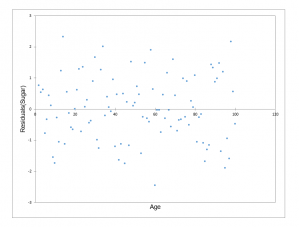Breusch-Pagan & White heteroscedasticity tests let you check if the residuals of a regression have changing variance. In Excel with the XLSTAT software.## What is heteroscedasticity?

The concept of heteroscedasticity - the opposite being homoscedasticity - is used in statistics, especially in the context of linear regression or for time series analysis, to describe the case where the variance of errors or the model is not the same for all observations, while often one of the basic assumption in modeling is that the variances are homogeneous and that the errors of the model are identically distributed.

In linear regression analysis, the fact that the errors of the model (also named residuals) are not homoskedastic has the consequence that the model coefficients estimated using ordinary least squares (OLS) are neither unbiased nor those with minimum variance. The estimation of their variance is not reliable.

## Testing for Heteroscedasticity

If it is suspected that the variances are not homogeneous (a representation of the residuals against the explanatory variables may reveal heteroscedasticity), it is therefore necessary to perform a test for heteroscedasticity. Several tests have been developed, with the following null and alternative hypotheses:

• H0 : The residuals are homoscedastic
• Ha : The residuals are heteroscedastic

XLSTAT now includes:

• Breusch-Pagan test
• White test and modified White test (Wooldridge)### analice sus datos con xlstat

prueba gratuita de 14 días

Incluido en